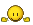# A nice logical challenge

#### c00l.d00d

Registered
Let´s see who gets this one right..You have 10 packages of cookies. In each package there are 10 cookies, where each cookie weighs 10 grams, totalling 100 grams in the package. But in one of 10 the packages, each cookie weighs 9 grams, totalling 90 grams.

Now, usings a scale like thisand weighing ONLY ONCE, how can you find the package that weighs 90 grams?

well if you weigh the first one and that is 90g you have found it. if is 100g you know the other one is 90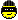well if you weigh the first one and that is 90g you have found it. if is 100g you know the other one is 90You must give an answer that guarantees which one is the 90g one...if you weigh one that is 100g, you won´t know which of the other nine is the 90g..:grinning:

I don't know if this counts, but you keep adding the packages one by one, rather than putting them all on the scale at the same time?

I don't know if this counts, but you keep adding the packages one by one, rather than putting them all on the scale at the same time?

That counts as more than one weighing..Because each time you add a package it´s a new weighing...

You must give an answer that guarantees which one is the 90g one...if you weigh one that is 100g, you won´t know which of the other nine is the 90g..:grinning:

ok then if we take from the first package one cookie, from the second 2 cookies and so on till we take from the 10th package 10 cookies we' ll have: 1+2+3+4+5+6+7+8+9+10=55 cookies in total

If we weigh them and each cookie weight 10g then we would have 55 x 10 = 550g

so we put them on the scale, see the result and we can understand from which package is the lighter cookie because par example if the result is 549g then we can understand that the lighter cookie was on the first package from where we took one cookie, if the result was 548g we assume that it was from the second package from where we took 2 cookies and so on...

i hope i didn't mix up but i think that's the right way to do itok then if we take from the first package one cookie, from the second 2 cookies and so on till we take from the 10th package 10 cookies we' ll have: 1+2+3+4+5+6+7+8+9+10=55 cookies in total

If we weigh them and each cookie weight 10g then we would have 55 x 10 = 550g

so we put them on the scale, see the result and we can understand from which package is the lighter cookie because par example if the result is 549g then we can understand that the lighter cookie was on the first package from where we took one cookie, if the result was 548g we assume that it was from the second package from where we took 2 cookies and so on...

i hope i didn't mix up but i think that's the right way to do it100% correct!:grinning::grinning::grinning:

100% correct!:grinning::grinning::grinning: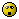am i winning something ???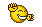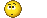am i winning something ???You can have the cookies...ok then if we take from the first package one cookie, from the second 2 cookies and so on till we take from the 10th package 10 cookies we' ll have: 1+2+3+4+5+6+7+8+9+10=55 cookies in total

If we weigh them and each cookie weight 10g then we would have 55 x 10 = 550g

so we put them on the scale, see the result and we can understand from which package is the lighter cookie because par example if the result is 549g then we can understand that the lighter cookie was on the first package from where we took one cookie, if the result was 548g we assume that it was from the second package from where we took 2 cookies and so on...

i hope i didn't mix up but i think that's the right way to do itsmart...